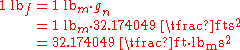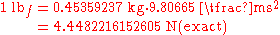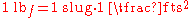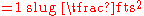xPound-forceEncyclopedia
The pound force is a unit
Units of measurement
A unit of measurement is a definite magnitude of a physical quantity, defined and adopted by convention and/or by law, that is used as a standard for measurement of the same physical quantity. Any other value of the physical quantity can be expressed as a simple multiple of the unit of...

of force
Force
In physics, a force is any influence that causes an object to undergo a change in speed, a change in direction, or a change in shape. In other words, a force is that which can cause an object with mass to change its velocity , i.e., to accelerate, or which can cause a flexible object to deform...

in some systems of measurement
Systems of measurement
A system of measurement is a set of units which can be used to specify anything which can be measured and were historically important, regulated and defined because of trade and internal commerce...

including English engineering units and British gravitational units.

## Definitions

The pound-force is equal to the gravitational force exerted on a mass of one avoirdupois pound on the surface of Earth
Earth
Earth is the third planet from the Sun, and the densest and fifth-largest of the eight planets in the Solar System. It is also the largest of the Solar System's four terrestrial planets...

. Since the 18th century, the unit has been used in low-precision measurements, for which small changes in Earth's gravity (which varies from place to place by up to half a percent) can safely be neglected.

The 20th century, however, brought the need for a more precise definition. A standardized value for acceleration due to gravity was therefore needed. Today, in accordance with the General Conference on Weights and Measures
General Conference on Weights and Measures
The General Conference on Weights and Measures is the English name of the Conférence générale des poids et mesures . It is one of the three organizations established to maintain the International System of Units under the terms of the Convention du Mètre of 1875...

, standard gravity
Standard gravity
Standard gravity, or standard acceleration due to free fall, usually denoted by g0 or gn, is the nominal acceleration of an object in a vacuum near the surface of the Earth. It is defined as precisely , or about...

is usually taken to be 9.80665 m/s2 (32.174 049 ft/s2).

The acceleration of the standard gravitational field
Standard gravity
Standard gravity, or standard acceleration due to free fall, usually denoted by g0 or gn, is the nominal acceleration of an object in a vacuum near the surface of the Earth. It is defined as precisely , or about...

(gn) and the international avoirdupois pound (lbm) define the pound-force as:This definition can be rephrased in terms of the slug. A slug has a mass unit equivalent of 32.174049 lbm. A pound-force is the amount of force required to accelerate a slug at a rate of 1 ft/s2, so:## Foot-pound-second systems of units

In some contexts, the term "pound" is used almost exclusively to refer to the unit of force and not the unit of mass. In those applications, the preferred unit of mass is the slug, i.e. lbf·s2/ft. In other contexts, the unit "pound" refers to a unit of mass
Pound (mass)
The pound or pound-mass is a unit of mass used in the Imperial, United States customary and other systems of measurement...

. In circumstances where there may otherwise be ambiguity, the symbols "lbf" and "lbm" and the terms "pounds-force" and "pounds-mass" can be used to distinguish.
In the gravitational systems, the weight
Weight
In science and engineering, the weight of an object is the force on the object due to gravity. Its magnitude , often denoted by an italic letter W, is the product of the mass m of the object and the magnitude of the local gravitational acceleration g; thus:...

of the mass unit (pound-mass) on Earth's surface is approximately equal to the force unit (pound-force). This is convenient because one pound mass exerts one pound force due to gravity. Note, however, unlike the other systems the force unit is not equal to the mass unit multiplied by the acceleration unit—the use of Newton's Second Law
Newton's laws of motion
Newton's laws of motion are three physical laws that form the basis for classical mechanics. They describe the relationship between the forces acting on a body and its motion due to those forces...

, F = m·a, requires another factor, gc, usually taken to be 32.174049 lbm·ft/lbf·s2 = 32.174049 lbm/slug.
"Absolute" systems are coherent systems of units: by using the slug as the unit of mass, the "engineering" FPS system avoids the need for such a constant. The SI
Si
Si, si, or SI may refer to :- Measurement, mathematics and science :* International System of Units , the modern international standard version of the metric system...

is an "absolute" metric system with kilogram and meter as base units.

• Poundal
Poundal
The poundal is a unit of force that is part of the foot-pound-second system of units, in Imperial units introduced in 1877, and is from the specialized subsystem of English Absolut ....

• Foot-pound (energy)
• Pound-mass
Pound (mass)
The pound or pound-mass is a unit of mass used in the Imperial, United States customary and other systems of measurement...

• Force
Force
In physics, a force is any influence that causes an object to undergo a change in speed, a change in direction, or a change in shape. In other words, a force is that which can cause an object with mass to change its velocity , i.e., to accelerate, or which can cause a flexible object to deform...

• mass
Mass
Mass can be defined as a quantitive measure of the resistance an object has to change in its velocity.In physics, mass commonly refers to any of the following three properties of matter, which have been shown experimentally to be equivalent:...

• Mass versus weight
Mass versus weight
In everyday usage, the mass of an object is often referred to as its weight though these are in fact different concepts and quantities. In scientific contexts, mass refers loosely to the amount of "matter" in an object , whereas weight refers to the force experienced by an object due to gravity...

for the difference between the two physical properties
• Weight
Weight
In science and engineering, the weight of an object is the force on the object due to gravity. Its magnitude , often denoted by an italic letter W, is the product of the mass m of the object and the magnitude of the local gravitational acceleration g; thus:...

for a more complete discussion of customary units of force and mass
• Pounds per square inch
Pounds per square inch
The pound per square inch or, more accurately, pound-force per square inch is a unit of pressure or of stress based on avoirdupois units...

, a unit of pressure
Pressure
Pressure is the force per unit area applied in a direction perpendicular to the surface of an object. Gauge pressure is the pressure relative to the local atmospheric or ambient pressure.- Definition :...

• Mass in general relativity
Mass in General Relativity
The concept of mass in general relativity is more complex than the concept of mass in special relativity. In fact, general relativity does not offer a single definition for the term mass, but offers several different definitions which are applicable under different circumstances...

• Mass in special relativity
Mass in special relativity
Mass in special relativity incorporates the general understandings from the concept of mass-energy equivalence. Added to this concept is an additional complication resulting from the fact that "mass" is defined in two different ways in special relativity: one way defines mass as an invariant...